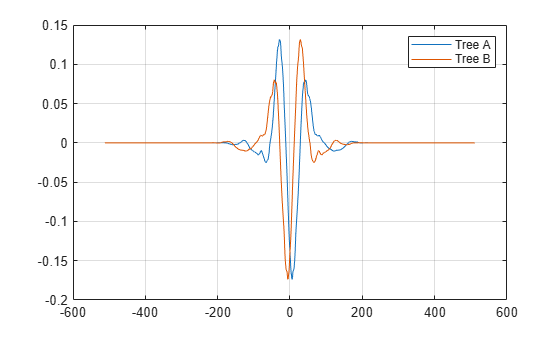# qorthwavf

Kingsbury Q-shift filters

## Syntax

``[LoDa,LoDb,HiDa,HiDb,LoRa,LoRb,HiRa,HiRb] = qorthwavf(num)``

## Description

example

````[LoDa,LoDb,HiDa,HiDb,LoRa,LoRb,HiRa,HiRb] = qorthwavf(num)` returns the Kingsbury Q-shift filters for the Q-shift complex dual-tree transform. The integer `num` refers to the number of nonzero coefficients (taps) in the filter. Valid options for `num` are 6, 10, 14, 16, and 18. All filters are of even lengths and the tree B filters are the time reverse of the tree A filters.```

## Examples

collapse all

Obtain the Q-shift filters for the case with 10 nonzero coefficients.

`[LoDa,LoDb,HiDa,HiDb,LoRa,LoRb,HiRa,HiRb] = qorthwavf(10);`

Use the `dwtfilterbank` function and create two discrete wavelet transform filter banks. Use the tree A analysis filters in the first filter bank, and the tree B analysis filters in the second filter bank.

```fbTreeA = dwtfilterbank('Wavelet','Custom',... 'CustomScalingFilter',LoDa,... 'CustomWaveletFilter',HiDa); fbTreeB = dwtfilterbank('Wavelet','Custom',... 'CustomScalingFilter',LoDb,... 'CustomWaveletFilter',HiDb);```

Plot the coarsest-scale wavelets of each filter bank.

```[psiA,t] = wavelets(fbTreeA); [psiB,~] = wavelets(fbTreeB); plot(t,psiA(end,:)) hold on plot(t,psiB(end,:)) grid on hold off legend('Tree A','Tree B')```Confirm both filter banks are orthogonal.

`isOrthogonal(fbTreeA)`
```ans = logical 1 ```
`isOrthogonal(fbTreeB)`
```ans = logical 1 ```

## Input Arguments

collapse all

Number of nonzero coefficients in the Kingsbury Q-shift filters, specified as one of the listed values.

## Output Arguments

collapse all

Tree A lowpass (scaling) analysis filter associated with the Q-shift filter, returned as a real-valued vector.

Tree B lowpass (scaling) analysis filter associated with the Q-shift filter, returned as a real-valued vector.

Tree A highpass (wavelet) analysis filter associated with the Q-shift filter, returned as a real-valued vector.

Tree B highpass (wavelet) analysis filter associated with the Q-shift filter, returned as a real-valued vector.

Tree A lowpass (scaling) synthesis filter associated with the Q-shift filter, returned as a real-valued vector.

Tree B lowpass (scaling) synthesis filter associated with the Q-shift filter, returned as a real-valued vector.

Tree A highpass (wavelet) synthesis filter associated with the Q-shift filter, returned as a real-valued vector.

Tree B highpass (wavelet) synthesis filter associated with the Q-shift filter, returned as a real-valued vector.

 Antonini, M., M. Barlaud, P. Mathieu, and I. Daubechies. “Image Coding Using Wavelet Transform.” IEEE Transactions on Image Processing 1, no. 2 (April 1992): 205–20. https://doi.org/10.1109/83.136597.

 Kingsbury, Nick. “Complex Wavelets for Shift Invariant Analysis and Filtering of Signals.” Applied and Computational Harmonic Analysis 10, no. 3 (May 2001): 234–53. https://doi.org/10.1006/acha.2000.0343.

 Le Gall, D., and A. Tabatabai. “Sub-Band Coding of Digital Images Using Symmetric Short Kernel Filters and Arithmetic Coding Techniques.” In ICASSP-88., International Conference on Acoustics, Speech, and Signal Processing, 761–64. New York, NY, USA: IEEE, 1988. https://doi.org/10.1109/ICASSP.1988.196696.